Translating to Word Statements

 Problem Set 1 Problem Set 2 Problem Set 3Select a problem set using the buttons above, then use your mouse or tab key to select a question. Fill in the blank with the correct answer for each problem. When you have answered all of the questions, ask Charlie how you did.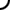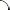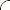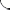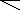Finish translating each mathematical expression into words. Fill in each blank with sum, difference, or is.   8 - 7 The of eight and seven. -5 + 3 The of negative five and three. x - 20 = 4 The difference of x and twenty four. 12 - y The of twelve and y. -2 + z = 6 The of negative two and z is six. w + 11 = 10 The sum of w and eleven ten.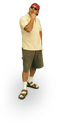Finish translating each mathematical expression into words. Fill in each blank with sum, difference, or is.   2 + 8 The of two and eight. x + 3 = 13 The of x and three is thirteen. -5 - y = 7 The difference of negative five and y seven. 9 - 2 The of nine and two. z - 1 = 50 The difference of z and one fifty. w - 6 The of w and six.Finish translating each mathematical expression into words. Fill in each blank with sum, difference, or is.   4 - x = 7 The of four and x is seven. y + 5 = 10 The sum of y and five ten. -11 - 6 The of negative eleven and six. z + 8 The of z and eight. w + 3 = 8 The sum of w and three eight. -1 + 4 The of negative one and four.©  2009  LarryPerezWeb Design:  Patrick Quigley